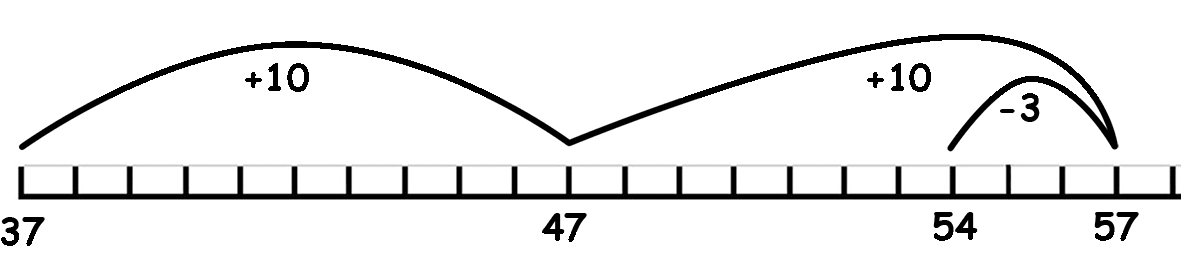Before we move on to written addition calculations it is useful to look at some basic methods of mental addition that you probably use all the time but have never thought about.

## Number Bonds (pairs that make up a number)

It is important for your child to know the number bonds that can be made by using the numbers 0 to 10. Begin with a target number of 10 and find all the pairs of numbers that make up 10. Say a number (0 to 10) and ask for the matching number to make 10. you say '4' - they say '6 ' - when they can do this easily, change the target number to 20 - you say '12' - they say '8' and so on. Then work on all the numbers up to 20. Knowing all the number bonds to 20 will really help when your child progresses to written addition and subtraction.

Number bonds for 100 are particularly useful - start with tens - you say '10' they say '90', then work in the 5's - you say '25' they say '75' and so on.

Practice number bonds with this online Number Bond Machine.

Or practice your mental addition with Math Trainer.## Partitioning (splitting up numbers)

More difficult numbers can be broken up into smaller numbers which are easier to manage.     73 is the same as 70 and 3 - 19 is the same as 10 and 9.

So if you need to add 73 + 19, split the numbers into 70+10+3+9, add the tens up and then the units. Do the same with larger numbers - 147 becomes 100+40+7.

## Rounding

Say you needed to add 37 and 9 - Round the 9 up to a 10 and add it to 37 to give 37+10=47, as 10 is 1 more than 9 you then need to subtract 1 from the total, 47-1=46.  So 37+9=46.

## Doubling and Halving

Practice doubling numbers up to 50 and halving numbers up to 100 - use partitioning to make the numbers easier (double the tens first and then double the units)
eg. for 27 -  20x2=40  7x2=14  40+14=54

If you need to add two similar numbers, say 33+35, you can double one of the numbers and then add or subtract the difference - 33x2=66 then 66+2=68 or 35x2=70 then 70-2=68

## Number Lines - counting on

### or     37+10=47    47+10=57     57-3=54For a printable page of blank number lines
or various number lines